Next: Vector Area Up: Vectors Previous: Vectors

## Vector Algebra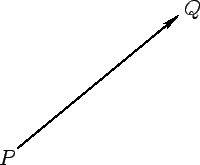In applied mathematics, physical quantities are (predominately) represented by two distinct classes of objects. Some quantities, denoted scalars, are represented by real numbers. Others, denoted vectors, are represented by directed line elements in space: e.g.,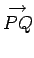in see Fig. 1. Note that line elements (and, therefore, vectors) are movable, and do not carry intrinsic position information: i.e., in Fig. 2,and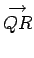are considered to be the same vector. In fact, vectors just possess a magnitude and a direction, whereas scalars possess a magnitude but no direction. By convention, vector quantities are denoted by bold-faced characters (e.g.,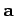) in typeset documents. Vector addition can be represented using a parallelogram: e.g.,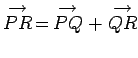in Fig. 2.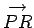is said to be the resultant ofand. Suppose that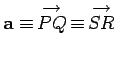,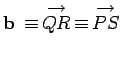, and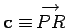. It follows, from Fig. 2, that vector addition is commutative: i.e.,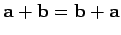(sinceis also the resultant ofand). It can also be shown that the associative law holds: i.e.,.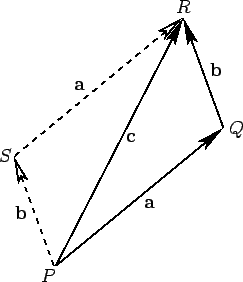There are two general approaches to vector analysis. The geometric approach is based on drawing line elements in space, and then making use of the theorems of Euclidian geometry. The coordinate approach assumes that space is defined by Cartesian coordinates, and uses these to characterize vectors. In Physics, we generally adopt the second approach, because it is far more convenient.

In the coordinate approach, a vector is denoted as the row matrix of its components along each of the Cartesian axes (the-,-, and-axes, say):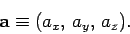(1)

Here,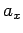is the-coordinate of the head'' of the vector minus the-coordinate of its tail,'' etc. If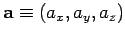and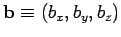then vector addition is defined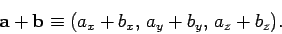(2)

Ifis a vector and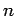is a scalar then the product of a scalar and a vector is defined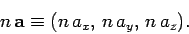(3)

The vectoris interpreted as a vector which points in the same direction as(or in the opposite direction, if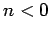), and is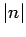times as long as. It is clear that vector algebra is distributive with respect to scalar multiplication: i.e.,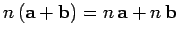.

Unit vectors can be defined in the-,-, and-directions as,, and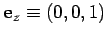. Any vector can be written in terms of these unit vectors: i.e.,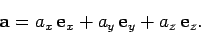(4)

In mathematical terminology, three vectors used in this manner form a basis of the vector space. If the three vectors are mutually perpendicular then they are termed orthogonal basis vectors. However, any set of three non-coplanar vectors can be used as basis vectors.

Examples of vectors in Physics are displacements from an origin,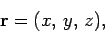(5)

and velocities,(6)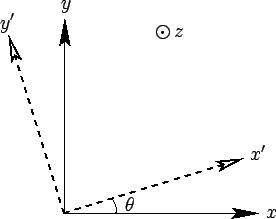Suppose that we transform to a new orthogonal basis, the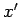-,-, and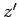-axes, which are related to the-,-, and-axes via a rotation through an anglearound the-axis--see Fig. 3. In the new basis, the coordinates of the general displacement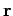from the origin are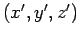. These coordinates are related to the previous coordinates via the transformation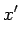(7)(8)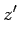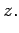(9)

Now, we do not need to change our notation for the displacement in the new basis. It is still denoted. The reason for this is that the magnitude and direction ofare independent of the choice of basis vectors. The coordinates ofdo depend on the choice of basis vectors. However, they must depend in a very specific manner [i.e., Eqs. (7)-(9)] which preserves the magnitude and direction of.

Since any vector can be represented as a displacement from an origin (this is just a special case of a directed line element), it follows that the components of a general vectormust transform in an similar manner to Eqs. (7)-(9). Thus,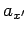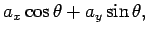(10)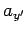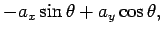(11)(12)

with analogous transformation rules for rotation about the- and-axes. In the coordinate approach, Eqs. (10)-(12) constitute the definition of a vector. The three quantities (,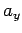,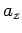) are the components of a vector provided that they transform under rotation like Eqs. (10)-(12). Conversely, (,,) cannot be the components of a vector if they do not transform like Eqs. (10)-(12). Scalar quantities are invariant under transformation. Thus, the individual components of a vector (, say) are real numbers, but they are not scalars. Displacement vectors, and all vectors derived from displacements, automatically satisfy Eqs. (10)-(12). There are, however, other physical quantities which have both magnitude and direction, but which are not obviously related to displacements. We need to check carefully to see whether these quantities are vectors.Next: Vector Area Up: Vectors Previous: Vectors
Richard Fitzpatrick 2007-07-14